﻿ Allee效应对第一个种群具有避难所的偏害系统的动力学行为影响 The Influence of the Allee Effect on the Dynamic Behavior of Two Species Amensalism System with a Refuge for the First Species

Vol. 08  No. 06 ( 2019 ), Article ID: 31062 , 15 pages
10.12677/AAM.2019.86135

The Influence of the Allee Effect on the Dynamic Behavior of Two Species Amensalism System with a Refuge for the First Species

Xiaoyan Huang, Fengde Chen

College of Mathematics and Computer Science, Fuzhou University, Fuzhou Fujian

Email: fdchen@fzu.edu.cn

Received: Jun. 11th, 2019; accepted: Jun. 21st, 2019; published: Jun. 28th, 2019ABSTRACT

In this paper, the Allee effect on the intrinsic growth rate is incorporate to the first species of two species amensalism model with a refuge for the first species. The existence and stability of the equilibria of the system are discussed. The study shows that after considering the Allee effect of the intrinsic growth rate of population, the number and the stability of equilibria of the system change with the change of the parameter and then the saddle-node bifurcation are generated.

Keywords:Amensalism, Refuge, Allee Effect, Bifurcation

Allee效应对第一个种群具有避难所的偏害系统的动力学行为影响

Email: fdchen@fzu.edu.cn1. 引言

2016年，Xie，Chen和He在文  提出了对第一个种群加入避难所以保护其免受第二个种群的破坏的偏害系统：

$\left\{\begin{array}{l}\frac{\text{d}x}{\text{d}t}={a}_{1}x\left(t\right)-{b}_{1}{x}^{2}\left(t\right)-{c}_{1}\left(1-k\right)x\left(t\right)y\left(t\right),\\ \frac{\text{d}y}{\text{d}t}={a}_{2}x\left(t\right)-{b}_{2}{y}^{2}\left(t\right).\end{array}$ (1.1)

${E}_{0}\left(0,0\right)$${E}_{1}\left(\frac{{a}_{1}}{{b}_{1}},0\right)$${E}_{2}\left(0,\frac{{a}_{2}}{{b}_{2}}\right)$，系统还可能存在的平衡点是 ${E}_{3}\left(\frac{{a}_{1}{b}_{2}-{a}_{2}{c}_{1}\left(1-k\right)}{{b}_{1}{b}_{2}},\frac{{a}_{2}}{{b}_{2}}\right)$。作者探讨了这四个平衡点的稳定性， ${E}_{0}\left(0,0\right)$${E}_{1}\left(\frac{{a}_{1}}{{b}_{1}},0\right)$ 均不稳定，若 $0，则 ${E}_{2}\left(0,\frac{{a}_{2}}{{b}_{2}}\right)$ 全局稳定；若 $1-\frac{{a}_{1}{b}_{2}}{{a}_{2}{c}_{1}}，则 ${E}_{3}$ 是唯一全局稳定的正平衡点。这一结果表明对于两种群偏害系统，如果避难所的容量足够大，使得第一个种群受到第二个种群的影响是有限的，则两个种群能稳定共存。反之，如果避难

$\frac{\text{d}N}{\text{d}t}=rN\left(1-\frac{N}{K}\right).$ (1.2)

(其中N是种群在t时刻的密度，r是内禀增长率，K是环境容纳量)只有唯一一个全局稳定的正平衡点 $N=K$，这个现象意味着种群能够长久生存下去。但是，随着人类对自然界的过度开发，越来越多的生物濒临灭绝，随着生物数量的减少，它们在择偶，抵御天敌，捕食等方面出现了极大的困难，所以，进一步降低了生物的出生率，这一现象在上个世纪30年代引起了学者Allee  的关注。后来，学者们(  -  )先后建立并研究了各种各样描述这种现象的生物系统，我们称之为具有Allee效应的种群系统。

2005年，Zhou，Liu和Wang在文  中提出了食饵种群的内禀增长率具有Allee效应的捕食–食饵模型：

$\left\{\begin{array}{l}\frac{\text{d}N}{\text{d}t}={r}_{1}N\left(\frac{N}{N+{A}_{1}}\right)-\epsilon PN,\\ \frac{\text{d}P}{\text{d}t}=-{r}_{2}P+\theta PN.\end{array}$ (1.3)

2016年，刘志广，张丰盘和尤春晓在文  中提出了下面食饵种群具有弱Allee效应的捕食–食饵模型：

$\left\{\begin{array}{l}\frac{\text{d}N}{\text{d}t}=N\left(\frac{rN}{A+N}-aN\right)-bNP,\\ \frac{\text{d}P}{\text{d}t}=cNP-dP.\end{array}$ (1.4)

$\left\{\begin{array}{l}\stackrel{˙}{x}=\frac{{a}_{1}x}{\beta +x}x-{b}_{1}{x}^{2}-{c}_{1}\left(1-k\right)xy,\\ \stackrel{˙}{y}={a}_{2}y-{b}_{2}{y}^{2}.\end{array}$ (1.5)

2. 系统(1.5)平衡点的存在

1) 当 ${a}_{1}<{a}^{\ast }$ 时，系统(1.5)没有正平衡点；

2) 当 ${a}_{1}={a}^{\ast }$ 时，系统(1.5)只有唯一一个正平衡点 ${E}^{*}\left({x}^{*},{y}^{*}\right)$

3) 当 ${a}_{1}>{a}^{\ast }$ 时，系统(1.5)有两个正平衡点 ${E}_{1}^{*}\left({x}_{1}^{*},{y}_{1}^{*}\right)$${E}_{2}^{*}\left({x}_{2}^{*},{y}_{2}^{*}\right)$

${a}^{*}=\frac{{\left(\sqrt{{b}_{1}{b}_{2}\beta }+\sqrt{{a}_{2}{c}_{1}\left(1-k\right)}\right)}^{2}}{{b}_{2}},$

${x}^{*}=\sqrt{\frac{{a}_{2}{c}_{1}\beta \left(1-k\right)}{{b}_{1}{b}_{2}}},{y}^{*}={y}_{1}^{*}={y}_{2}^{*}=\frac{{a}_{2}}{{b}_{2}},$ (2.1)

${x}_{1}^{*}=\frac{{a}_{1}{b}_{2}-{b}_{1}{b}_{2}\beta -{a}_{2}{c}_{1}\left(1-k\right)-\sqrt{{\left[{b}_{1}{b}_{2}\beta +{a}_{2}{c}_{1}\left(1-k\right)-{a}_{1}{b}_{2}\right]}^{2}-4{a}_{2}{b}_{1}{b}_{2}{c}_{1}\beta \left(1-k\right)}}{2{b}_{1}{b}_{2}},$ (2.2)

${x}_{2}^{*}=\frac{{a}_{1}{b}_{2}-{b}_{1}{b}_{2}\beta -{a}_{2}{c}_{1}\left(1-k\right)+\sqrt{{\left[{b}_{1}{b}_{2}\beta +{a}_{2}{c}_{1}\left(1-k\right)-{a}_{1}{b}_{2}\right]}^{2}-4{a}_{2}{b}_{1}{b}_{2}{c}_{1}\beta \left(1-k\right)}}{2{b}_{1}{b}_{2}},$ (2.3)

${x}_{1}^{*}<{x}^{*}<{x}_{2}^{*}.$

$\left\{\begin{array}{l}\frac{{a}_{1}{x}^{2}}{\beta +x}-{b}_{1}{x}^{2}-{c}_{1}\left(1-k\right)xy=0,\\ {a}_{2}y-{b}_{2}{y}^{2}=0.\end{array}$ (2.4)

$\frac{{a}_{1}x}{\beta +x}x-{b}_{1}{x}^{2}-{c}_{1}\left(1-k\right)\frac{{a}_{2}}{{b}_{2}}x=0.$ (2.5)

${b}_{1}{b}_{2}{x}^{2}+\left[{b}_{1}{b}_{2}\beta +{a}_{2}{c}_{1}\left(1-k\right)-{a}_{1}{b}_{2}\right]x+{a}_{2}{c}_{1}\beta \left(1-k\right)=0,$ (2.6)

(2.6)的判别式是

$\Delta ={\left[{b}_{1}{b}_{2}\beta +{a}_{2}{c}_{1}\left(1-k\right)-{a}_{1}{b}_{2}\right]}^{2}-4{a}_{2}{b}_{1}{b}_{2}{c}_{1}\beta \left(1-k\right)$

$A{x}^{2}+Bx+C=0.$ (2.7)

$A={b}_{1}{b}_{2}>0,B={b}_{1}{b}_{2}\beta +{a}_{2}{c}_{1}\left(1-k\right)-{a}_{1}{b}_{2},C={a}_{2}{c}_{1}\beta \left(1-k\right)>0.$

1) 当 $\Delta <0$，此时通过计算得到 ${a}^{**}<{a}_{1}<{a}^{*}$，其中 ${a}^{**}=\frac{{\left(\sqrt{{b}_{1}{b}_{2}\beta }-\sqrt{{a}_{2}{c}_{1}\left(1-k\right)}\right)}^{2}}{{b}_{2}}$

${a}^{*}=\frac{{\left(\sqrt{{b}_{1}{b}_{2}\beta }+\sqrt{{a}_{2}{c}_{1}\left(1-k\right)}\right)}^{2}}{{b}_{2}}$。则(2.6)没有实数根，即系统(1.5)没有正平衡点。

2) 当 $\Delta =0$，此时 ${a}_{1}={a}^{**}$ 或者 ${a}_{1}={a}^{*}$，则(2.6)有唯一一个实数根 ${x}^{*}$

a) 若 ${a}_{1}={a}^{**}$，则(2.6)化为

${\left(\sqrt{{b}_{1}{b}_{2}}{x}^{*}+\sqrt{{a}_{2}{c}_{1}\beta \left(1-k\right)}\right)}^{2}=0,$

${x}^{*}=-\sqrt{\frac{{a}_{2}{c}_{1}\beta \left(1-k\right)}{{b}_{1}{b}_{2}}}<0.$

b) 若 ${a}_{1}={a}^{*}$，(2.6)化为

${\left(\sqrt{{b}_{1}{b}_{2}}{x}^{*}-\sqrt{{a}_{2}{c}_{1}\beta \left(1-k\right)}\right)}^{2}=0,$

${x}^{*}=\sqrt{\frac{{a}_{2}{c}_{1}\beta \left(1-k\right)}{{b}_{1}{b}_{2}}}>0.$

3) 当 $\Delta >0$，计算得 $a>{a}^{*}$ 或者 $a<{a}^{**}$，此时(2.6)有两个不同的实数根 ${x}_{1}^{*},{x}_{2}^{*}$

a) 若 $a>{a}^{*}$，此时 $B<0$，结合(2.7)，由韦达定理得到

$\begin{array}{l}{x}_{1}^{*}+{x}_{2}^{*}=-\frac{B}{A}>0,\\ {x}_{1}^{*}{x}_{2}^{*}=\frac{C}{A}>0.\end{array}$

${x}_{2}^{*}>\frac{{a}_{1}{b}_{2}-{b}_{1}{b}_{2}\beta -{a}_{2}{c}_{1}\left(1-k\right)}{2{b}_{1}{b}_{2}}>\sqrt{\frac{{a}_{2}{c}_{1}\beta \left(1-k\right)}{{b}_{1}{b}_{2}}}={x}^{*}.$

${x}_{1}^{*}{x}_{2}^{*}=\frac{C}{A}=\frac{{a}_{2}{c}_{1}\beta \left(1-k\right)}{{b}_{1}{b}_{2}},$

${x}_{1}^{*}=\frac{{a}_{2}{c}_{1}\beta \left(1-k\right)}{{b}_{1}{b}_{2}}\frac{1}{{x}_{2}^{*}}<\sqrt{\frac{{a}_{2}{c}_{1}\beta \left(1-k\right)}{{b}_{1}{b}_{2}}}={x}^{*}.$

b) 若 ${a}_{1}<{a}^{**}$，这时候 $B>0$，根据(2.6)和韦达定理

$\begin{array}{l}{x}_{1}^{*}+{x}_{2}^{*}=-\frac{B}{A}<0,\\ {x}_{1}^{*}{x}_{2}^{*}=\frac{C}{A}>0.\end{array}$

3. 系统(1.5)平衡点的稳定性

$J\left(x,y\right)=\left(\begin{array}{cc}\frac{{a}_{1}x\left(x+2\beta \right)}{{\left(x+\beta \right)}^{2}}-2{b}_{1}x-{c}_{1}\left(1-k\right)y& -{c}_{1}\left(1-k\right)x\\ 0& {a}_{2}-2{b}_{2}y\end{array}\right)$(3.1)

${\lambda }_{2}={a}_{2}$。所以 ${E}_{0}$ 是非双曲的，不能直接判断其稳定性。接下来我们利用文  定理7.1来讨论其稳定性。

$\begin{array}{l}\frac{\text{d}x}{\text{d}t}=-{c}_{1}\left(1-k\right)xy+\left(\frac{{a}_{1}}{\beta }-{b}_{1}\right){x}^{2}-\frac{{a}_{1}}{{\beta }^{2}}{x}^{3}+\frac{{a}_{1}}{{\beta }^{3}}{x}^{4}-\frac{{a}_{1}}{{\beta }^{4}}{x}^{5}+{P}_{1}\left(x,y\right),\\ \frac{\text{d}y}{\text{d}t}={a}_{2}y-{b}_{2}{y}^{2}.\end{array}$ (3.2)

$\begin{array}{l}\frac{\text{d}x}{\text{d}\tau }=-\frac{{c}_{1}\left(1-k\right)}{{a}_{2}}xy+\frac{1}{a{}_{2}}\left(\frac{{a}_{1}}{\beta }-{b}_{1}\right){x}^{2}-\frac{{a}_{1}}{{a}_{2}{\beta }^{2}}{x}^{3}+\frac{{a}_{1}}{{a}_{2}{\beta }^{3}}{x}^{4}-\frac{{a}_{1}}{{a}_{2}{\beta }^{4}}{x}^{5}+{P}_{2}\left(x,y\right),\\ \frac{\text{d}y}{\text{d}\tau }=y-\frac{{b}_{2}}{{a}_{2}}{y}^{2}.\end{array}$ (3.3)

$\frac{\text{d}x}{\text{d}\tau }=\frac{1}{{a}_{2}}\left(\frac{{a}_{1}}{\beta }-{b}_{1}\right){x}^{2}-\frac{{a}_{1}}{{a}_{2}{\beta }^{2}}{x}^{3}+\frac{{a}_{1}}{{a}_{2}{\beta }^{3}}{x}^{4}-\cdots .$ (3.4)

$\frac{{a}_{1}}{\beta }-{b}_{1}\ne 0$ 时，则 $m=2$${a}_{m}=\frac{1}{{a}_{2}}\left(\frac{{a}_{1}}{\beta }-{b}_{1}\right)\ne 0$。根据  定理7.1可知， ${E}_{0}\left(0,0\right)$ 是鞍结点。

$\frac{{a}_{1}}{\beta }-{b}_{1}=0$ 时，则 $m=3$${a}_{m}=-\frac{{a}_{1}}{{a}_{2}{\beta }^{2}}<0$。根据  定理7.1可知， ${E}_{0}\left(0,0\right)$ 是鞍点。

$J\left({E}_{1}\right)=\left(\begin{array}{cc}-{c}_{1}\left(1-k\right)\frac{{a}_{2}}{{b}_{2}}& 0\\ 0& -{a}_{2}\end{array}\right),$

${E}_{2}$ 存在，则系统(1.5)在 ${E}_{2}$ 处的雅克比矩阵为

$J\left({E}_{2}\right)=\left(\begin{array}{cc}-\frac{{\left({a}_{1}-{b}_{1}\beta \right)}^{2}}{{a}_{1}}& -{c}_{1}\left(1-k\right)\left(\frac{{a}_{1}}{{b}_{1}}-\beta \right)\\ 0& {a}_{2}\end{array}\right),$

${a}_{1}-{b}_{1}\beta >0$，则此时有两个特征值分别是 ${\lambda }_{1}=-\frac{{\left({a}_{1}-{b}_{1}\beta \right)}^{2}}{{a}_{1}}<0$${\lambda }_{2}={a}_{2}>0$，所以 ${E}_{2}$ 是鞍点。若 ${a}_{1}-{b}_{1}\beta =0$，则 $\frac{{a}_{1}}{{b}_{1}}-\beta =0$，此时 ${E}_{2}$${E}_{0}$ 重合，所以 ${E}_{2}$ 仍然是鞍点。

$J\left(x,y\right)=\left(\begin{array}{cc}{F}_{1}& -{c}_{1}\left(1-k\right)x\\ 0& -{a}_{2}\end{array}\right),$

$\begin{array}{c}{F}_{1}=\frac{{a}_{1}x\left(x+2\beta \right)}{{\left(x+\beta \right)}^{2}}-2{b}_{1}x-{c}_{1}\left(1-k\right)y\\ =\frac{{a}_{1}x}{\beta +x}-{b}_{1}x-{c}_{1}\left(1-k\right)y+\frac{{a}_{1}\beta x}{{\left(\beta +x\right)}^{2}}-{b}_{1}x\\ =\frac{{a}_{1}\beta x}{{\left(\beta +x\right)}^{2}}-{b}_{1}x\\ =x\left[\frac{{a}_{1}\beta }{{\left(\beta +x\right)}^{2}}-{b}_{1}\right].\end{array}$

$\begin{array}{l}\left(\sqrt{\frac{{a}_{1}\beta }{{b}_{1}}}-\beta \right)-\sqrt{\frac{{a}_{2}{c}_{1}\beta \left(1-k\right)}{{b}_{1}{b}_{2}}}=\sqrt{\frac{{a}_{1}{b}_{2}\beta }{{b}_{1}{b}_{2}}}-\sqrt{\frac{{b}_{1}{b}_{2}{\beta }^{2}}{{b}_{1}{b}_{2}}}-\sqrt{\frac{{a}_{2}{c}_{1}\beta \left(1-k\right)}{{b}_{1}{b}_{2}}}\\ \text{\hspace{0.17em}}\text{\hspace{0.17em}}\text{\hspace{0.17em}}\text{\hspace{0.17em}}\text{\hspace{0.17em}}\text{\hspace{0.17em}}\text{\hspace{0.17em}}\text{\hspace{0.17em}}\text{\hspace{0.17em}}\text{\hspace{0.17em}}\text{\hspace{0.17em}}\text{\hspace{0.17em}}\text{\hspace{0.17em}}\text{\hspace{0.17em}}\text{\hspace{0.17em}}\text{\hspace{0.17em}}\text{\hspace{0.17em}}\text{\hspace{0.17em}}\text{\hspace{0.17em}}\text{\hspace{0.17em}}\text{\hspace{0.17em}}\text{\hspace{0.17em}}\text{\hspace{0.17em}}\text{\hspace{0.17em}}\text{\hspace{0.17em}}\text{\hspace{0.17em}}\text{\hspace{0.17em}}\text{\hspace{0.17em}}\text{\hspace{0.17em}}\text{\hspace{0.17em}}\text{\hspace{0.17em}}\text{\hspace{0.17em}}\text{\hspace{0.17em}}\text{\hspace{0.17em}}\text{\hspace{0.17em}}\text{\hspace{0.17em}}\text{\hspace{0.17em}}\text{\hspace{0.17em}}\text{\hspace{0.17em}}=\sqrt{\frac{{b}_{1}{b}_{2}{\beta }^{2}}{{b}_{1}{b}_{2}}+\frac{{a}_{2}{c}_{1}\beta \left(1-k\right)}{{b}_{1}{b}_{2}}+\frac{2\beta \sqrt{{a}_{2}{b}_{1}{b}_{2}{c}_{1}\beta \left(1-k\right)}}{{b}_{1}{b}_{2}}}-\sqrt{\frac{{b}_{1}{b}_{2}{\beta }^{2}}{{b}_{1}{b}_{2}}}-\sqrt{\frac{{a}_{2}{c}_{1}\beta \left(1-k\right)}{{b}_{1}{b}_{2}}}\\ \text{\hspace{0.17em}}\text{\hspace{0.17em}}\text{\hspace{0.17em}}\text{\hspace{0.17em}}\text{\hspace{0.17em}}\text{\hspace{0.17em}}\text{\hspace{0.17em}}\text{\hspace{0.17em}}\text{\hspace{0.17em}}\text{\hspace{0.17em}}\text{\hspace{0.17em}}\text{\hspace{0.17em}}\text{\hspace{0.17em}}\text{\hspace{0.17em}}\text{\hspace{0.17em}}\text{\hspace{0.17em}}\text{\hspace{0.17em}}\text{\hspace{0.17em}}\text{\hspace{0.17em}}\text{\hspace{0.17em}}\text{\hspace{0.17em}}\text{\hspace{0.17em}}\text{\hspace{0.17em}}\text{\hspace{0.17em}}\text{\hspace{0.17em}}\text{\hspace{0.17em}}\text{\hspace{0.17em}}\text{\hspace{0.17em}}\text{\hspace{0.17em}}\text{\hspace{0.17em}}\text{\hspace{0.17em}}\text{\hspace{0.17em}}\text{\hspace{0.17em}}\text{\hspace{0.17em}}\text{\hspace{0.17em}}\text{\hspace{0.17em}}\text{\hspace{0.17em}}\text{\hspace{0.17em}}\text{\hspace{0.17em}}=0.\end{array}$

${F}_{1}\left({x}^{*}\right)={x}^{*}g\left({x}^{*}\right)=0$，所以 ${E}^{*}$ 是非双曲的奇点，无法直接判断其稳定性。接下来我们利用文  中的定理7.1来判断其稳定性。

$\begin{array}{l}\frac{\text{d}X}{\text{d}t}={d}_{01}Y+{d}_{02}{X}^{2}+{d}_{03}XY+{d}_{04}{X}^{3}+{d}_{05}{X}^{4}+{d}_{06}{X}^{5}+{Q}_{1}\left(X,Y\right),\\ \frac{\text{d}Y}{\text{d}t}=-{a}_{2}Y-{b}_{2}{Y}^{2}.\end{array}$ (3.5)

$\begin{array}{l}\frac{\text{d}u}{\text{d}t}={d}_{02}{\left(u-\frac{{d}_{01}}{{a}_{2}}v\right)}^{2}+{d}_{03}\left(u-\frac{{d}_{01}}{{a}_{2}}v\right)v-\frac{{d}_{01}{b}_{2}}{{a}_{2}}{v}^{2}+{d}_{04}{\left(u-\frac{{d}_{01}}{{a}_{2}}v\right)}^{3}\\ \text{\hspace{0.17em}}\text{\hspace{0.17em}}\text{\hspace{0.17em}}\text{\hspace{0.17em}}\text{\hspace{0.17em}}\text{\hspace{0.17em}}\text{\hspace{0.17em}}\text{\hspace{0.17em}}+{d}_{05}{\left(u-\frac{{d}_{01}}{{a}_{2}}v\right)}^{4}+{d}_{06}{\left(u-\frac{{d}_{01}}{{a}_{2}}v\right)}^{5}+{Q}_{2}\left(u,v\right),\\ \frac{\text{d}v}{\text{d}t}=-{a}_{2}v-{b}_{2}{v}^{2}.\end{array}$ (3.6)

$\begin{array}{l}\frac{\text{d}u}{\text{d}\tau }=-\frac{{d}_{02}}{{a}_{2}}{\left(u-\frac{{d}_{01}}{{a}_{2}}v\right)}^{2}-\frac{{d}_{03}}{{a}_{2}}\left(u-\frac{{d}_{01}}{{a}_{2}}v\right)v+\frac{{d}_{01}{b}_{2}}{{a}_{2}^{2}}{v}^{2}-\frac{{d}_{04}}{{a}_{2}}{\left(u-\frac{{d}_{01}}{{a}_{2}}v\right)}^{3}\\ \text{ }\text{\hspace{0.17em}}\text{\hspace{0.17em}}\text{\hspace{0.17em}}\text{\hspace{0.17em}}\text{\hspace{0.17em}}\text{\hspace{0.17em}}\text{\hspace{0.17em}}-\frac{{d}_{05}}{{a}_{2}}{\left(u-\frac{{d}_{01}}{{a}_{2}}v\right)}^{4}-\frac{{d}_{06}}{{a}_{2}}{\left(u-\frac{{d}_{01}}{{a}_{2}}v\right)}^{5}+{Q}_{3}\left(u,v\right),\\ \frac{\text{d}v}{\text{d}\tau }=v+\frac{{b}_{2}}{{a}_{2}}{v}^{2}.\end{array}$ (3.7)

$v+\frac{{b}_{2}}{{a}_{2}}{v}^{2}=0$ 解得隐函数 $v=\phi \left(u\right)=0$，从而得到

$\frac{\text{d}u}{\text{d}\tau }=-\frac{{d}_{02}}{{a}_{2}}{u}^{2}-\frac{{d}_{04}}{{a}_{2}}{u}^{3}-\cdots ,$

$\begin{array}{c}\stackrel{˙}{V}=\stackrel{˙}{x}+\left(y-{y}^{*}\right)\frac{\stackrel{˙}{y}}{y}\\ =\frac{{a}_{1}{x}^{2}}{\beta +x}-{b}_{1}{x}^{2}-{c}_{1}\left(1-k\right)xy+\left(y-\frac{{a}_{2}}{{b}_{2}}\right)\left({a}_{2}-{b}_{2}y\right)\\ =\left[\frac{{a}_{1}x}{\beta +x}-{c}_{1}\left(1-k\right)y\right]x-{b}_{1}{x}^{2}-\frac{1}{{b}_{2}}{\left({a}_{2}-{b}_{2}y\right)}^{2}\\ \le \left[\frac{{a}_{1}x}{\beta +x}-{c}_{1}\left(1-k\right)\left(\frac{{a}_{2}}{{b}_{2}}-\epsilon \right)\right]x-{b}_{1}{x}^{2}-\frac{1}{{b}_{2}}{\left({a}_{2}-{b}_{2}y\right)}^{2}\\ =\left\{\frac{\left[{a}_{1}-{c}_{1}\left(1-k\right)\left(\frac{{a}_{2}}{{b}_{2}}-\epsilon \right)\right]x-{c}_{1}\beta \left(1-k\right)\left(\frac{{a}_{2}}{{b}_{2}}-\epsilon \right)}{\beta +x}\right\}x-{b}_{1}{x}^{2}-\frac{1}{{b}_{2}}{\left({a}_{2}-{b}_{2}y\right)}^{2}.\end{array}$

4. 系统(1.5)的分支现象

${a}_{1}={a}_{SN}=\frac{{\left(\sqrt{{b}_{1}{b}_{2}\beta }+\sqrt{{a}_{2}{c}_{1}\left(1-k\right)}\right)}^{2}}{{b}_{2}},$ (4.1)

$J\left({E}^{*},{a}_{SN}\right)=\left(\begin{array}{cc}0& -{c}_{1}\left(1-k\right){x}^{*}\\ 0& -{a}_{2}\end{array}\right).$

$V=\left(\begin{array}{c}{V}_{1}\\ {V}_{2}\end{array}\right)=\left(\begin{array}{c}1\\ 0\end{array}\right),$ (4.2)

$W=\left(\begin{array}{c}{W}_{1}\\ {W}_{2}\end{array}\right)=\left(\begin{array}{c}1\\ -\frac{{c}_{1}\left(1-k\right){x}^{*}}{{a}_{2}}\end{array}\right).$ (4.3)

${F}_{{a}_{1}}\left({E}^{*};{a}_{SN}\right)={\left(\begin{array}{c}\frac{{x}^{2}}{\beta +x}\\ 0\end{array}\right)}_{x={x}^{*}}=\left(\begin{array}{c}\frac{{x}^{*2}}{\beta +{x}^{*}}\\ 0\end{array}\right),$ (4.4)

$\begin{array}{c}{D}^{2}F\left({E}^{*};{a}_{SN}\right)\left(V,V\right)={\left(\begin{array}{c}\frac{{\partial }^{2}{F}_{1}}{\partial {x}^{2}}{V}_{1}^{2}+2\frac{{\partial }^{2}{F}_{1}}{\partial x\partial y}{V}_{1}{V}_{2}+\frac{{\partial }^{2}{F}_{1}}{\partial {y}^{2}}{V}_{2}^{2}\\ \frac{{\partial }^{2}{F}_{2}}{\partial {x}^{2}}{V}_{1}^{2}+2\frac{{\partial }^{2}{F}_{2}}{\partial x\partial y}{V}_{1}{V}_{2}+\frac{{\partial }^{2}{F}_{2}}{\partial {y}^{2}}{V}_{2}^{2}\end{array}\right)}_{\left({E}^{*},{a}_{SN}\right)}\\ =\left(\begin{array}{c}\frac{2{a}_{1}{\beta }^{2}}{{\left(\beta +{x}^{*}\right)}^{3}}-2{b}_{1}\\ 0\end{array}\right).\end{array}$ (4.5)

${W}^{\text{T}}{F}_{{a}_{1}}\left({E}^{*};{a}_{SN}\right)=\frac{{x}^{*2}}{\beta +{x}^{*}}\ne 0,$ (4.6)

${W}^{\text{T}}\left[{D}^{2}F\left({E}^{*};{a}_{SN}\right)\left(V,V\right)\right]=\frac{2{a}_{1}{\beta }^{2}}{{\left(\beta +{x}^{*}\right)}^{3}}-2{b}_{1}\ne 0.$ (4.7)

5. 系统(1.5)数值模拟

1) a) 我们取值 ${a}_{1}={a}_{2}={b}_{1}={b}_{2}=1$$\beta ={c}_{1}=k=\frac{1}{2}$，此时 $\frac{{a}_{1}}{\beta }-{b}_{1}=1>0$，由前面分析知 ${E}_{0}\left(0,0\right)$ 是鞍结点， ${E}_{1}\left(0,1\right)$ 是稳定的结点， ${E}_{2}\left(\frac{1}{2},0\right)$ 是鞍点。图1所示。b) 取值 ${a}_{1}={a}_{2}={b}_{1}={b}_{2}=\beta =1$${c}_{1}=k=\frac{1}{2}$，此时 $\frac{{a}_{1}}{\beta }-{b}_{1}=0$。则 ${E}_{0}\left(0,0\right)$ 是鞍点， ${E}_{1}\left(0,1\right)$ 是稳定的结点，此时 ${E}_{2}$${E}_{0}$ 重合。图2所示。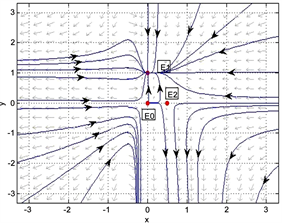Figure 1. Dynamics behaviors of the system (1.5), the parameters $\left({a}_{1},{a}_{2},{b}_{1},{b}_{2},{c}_{1},k,\beta \right)=\left(1,1,1,1,\frac{1}{2},\frac{1}{2},1\right)$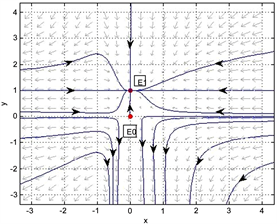Figure 2. Dynamics behaviors of the system (1.5), the parameters $\left({a}_{1},{a}_{2},{b}_{1},{b}_{2},{c}_{1},k,\beta \right)=\left(1,1,1,1,\frac{1}{2},\frac{1}{2},\frac{1}{2}\right)$

2) a) 我们取值 ${a}_{1}={a}_{2}={b}_{1}={b}_{2}=1$$\beta =\frac{1}{4}$${c}_{1}=k=\frac{1}{2}$，此时 $\frac{{\left(\sqrt{{b}_{1}{b}_{2}\beta }+\sqrt{{a}_{2}{c}_{1}\left(1-k\right)}\right)}^{2}}{{b}_{2}}=1={a}_{1}$，由定理2.1知，系统(1.5)只有唯一一个正平衡点 ${E}^{*}\left(\frac{1}{4},1\right)$，且该点是鞍结点。图3所示。b) 我们取值 ${a}_{1}=2$ , ${a}_{2}={b}_{1}={b}_{2}=1$$\beta =\frac{1}{4}$${c}_{1}=k=\frac{1}{2}$，此时 $\frac{{\left(\sqrt{{b}_{1}{b}_{2}\beta }+\sqrt{{a}_{2}{c}_{1}\left(1-k\right)}\right)}^{2}}{{b}_{2}}=1<{a}_{1}=2$，由定理2.1知，系统(1.5)有两个正平衡点 ${E}_{1}^{*}\left(\frac{3-2\sqrt{2}}{4},1\right)$${E}_{2}^{*}\left(\frac{3+2\sqrt{2}}{4},1\right)$，其中 ${E}_{1}^{*}$ 是鞍点， ${E}_{2}^{*}$ 是稳定的结点。图4所示。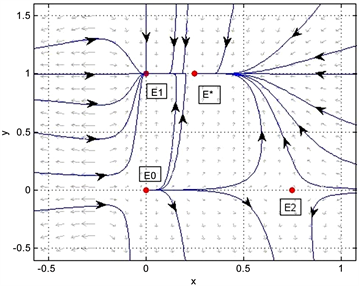Figure 3. Dynamics behaviors of the system (1.5), the parameters $\left({a}_{1},{a}_{2},{b}_{1},{b}_{2},{c}_{1},k,\beta \right)=\left(1,1,1,1,\frac{1}{2},\frac{1}{2},\frac{1}{4}\right)$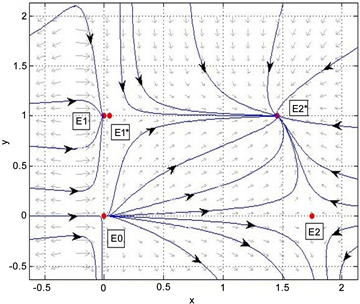Figure 4. Dynamics behaviors of the system (1.5), the parameters $\left({a}_{1},{a}_{2},{b}_{1},{b}_{2},{c}_{1},k,\beta \right)=\left(2,1,1,1,\frac{1}{2},\frac{1}{2},\frac{1}{4}\right)$

3) 我们取值 ${a}_{1}={a}_{2}={b}_{1}={b}_{2}=\beta =1$${c}_{1}=2$$k=\frac{1}{4}$，此时有 $1-\frac{{a}_{1}{b}_{2}}{{a}_{2}{c}_{1}}=\frac{1}{2}>k=\frac{1}{4}$，由定理3.1知， ${E}_{1}\left(0,1\right)$ 全局稳定。图5所示，红黄蓝绿色线条分别取初值 $\left(2.8,1\right)$$\left(0.5,0.7\right)$$\left(1.3,2.5\right)$$\left(0.2,1.6\right)$Figure 5. ${E}_{1}$ is globally stable when the parameters $\left(\beta ,k\right)=\left(1,\frac{1}{4}\right)$ of the system (1.5)

4) 我们取值 ${a}_{1}={a}_{2}={b}_{1}={b}_{2}=1$${c}_{1}=2$$k=\frac{1}{4}$，现在取一组固定初值 $\left(1.3,2.5\right)$，红蓝绿色线条分别取 $\beta =0.02,1.8,4.2$，则此时 ${E}_{1}\left(0,1\right)$ 是全局稳定，图6所示。我们发现Allee常数对系统(1.5)的第二个种群无明显影响。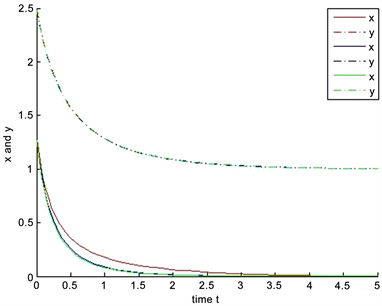Figure 6. The influence of the parameters $\beta =\left(0.02,1.8,4.2\right)$ of the system (1.5) on the speed at solution tends to $\left(0,1\right)$

6. 小结

Allee效应使得偏害系统的平衡点个数增加，并且平衡点的稳定性比较复杂(表1)：Table 1. The existence and stability of the equilibrium point of the system (1.5)

7. 讨论

1) 系统(1.5)存在六个平衡点(包括三个边界平衡点和三个正平衡点)，而系统(1.1)只有四个平衡点(包括三个边界平衡点和一个正平衡点)。这是因为我们在第一个种群的内禀增长率上考虑了Allee效应，使得系统的平衡点个数增加。

2) 随着平衡点个数的增加，系统(1.5)的动力学行为变得更加复杂，例如：产生了鞍结分支。

3) 在第一个种群的内禀增长率上考虑了Allee效应，使得第一个种群满足一定条件下最终会趋于绝灭，并通过数值模拟发现在相同初值的情况下，随着Allee效应的增大，第一个种群绝灭的速度越快。说明了Allee效应不利于种群的生长，这是因为Allee效应会降低种群的出生率。

The Influence of the Allee Effect on the Dynamic Behavior of Two Species Amensalism System with a Refuge for the First Species[J]. 应用数学进展, 2019, 08(06): 1166-1180. https://doi.org/10.12677/AAM.2019.86135

1. 1. 孙广才. 两种群偏害作用的系统的定性分析[J]. 佳木斯大学学报(自然科学版), 2003, 21(3): 284-286.

2. 2. 祝占法, 陈巧灵. 偏害关系的Lotka-Volterra系统的数学研究[J]. 鸡西大学学报, 2008, 8(5): 100-101.

3. 3. 熊焕焕, 王斌斌, 张海亮. 鱼群偏害共生动力学系统的稳定性分析[J]. 应用数学进展, 2016, 5(2): 255-261.

4. 4. Xie, X.D., Chen, F.D. and He, M.X. (2016) Dynamic Behaviors of Two Species Amensalism Model with a Cover for the First Species. Journal of Mathematics and Computer Science, 16, 395-401. https://doi.org/10.22436/jmcs.016.03.09

5. 5. Wu, R.X. (2016) A Two Species Amensalism Model with Non-Monotonic Functional Response. Communications in Mathematical Biology Neuroscience, 2016, Article ID: 19.

6. 6. Wu, R.X., Zhao, L. and Lin, Q.X. (2016) Stability Analysis of a Two Species Amensalism Model with Holling II Functional Response and a Cover for the First Species. Journal of Nonlinear Functional Analysis, 2016, Article ID: 46.

7. 7. Lei, C.Q. (2018) Dynamic Behaviors of a Stage Structure Amensalism System with a Cover for the First Species. Advances in Difference Equations, 2018, 272. https://doi.org/10.1186/s13662-018-1729-1

8. 8. Liu, Y., Zhao, L., Huang, X.Y. and Deng, H. (2018) Stability and Bifurcation Analysis of Two Species Amensalism Model with Michealis-Menten Type Harvesting and a Cover for the First Species. Advances in Difference Equations, 2018, 295. https://doi.org/10.1186/s13662-018-1752-2

9. 9. Chen, B. (2018) Dynamic Behaviors of a Non-Selective Harvesting Lotka-Volterra Amensalism Model Incorporating Partial Closure for the Populations. Advances in Difference Equations, 2018, 111. https://doi.org/10.1186/s13662-018-1555-5

10. 10. Moitri, S., Banerjee, M. and Morozov, A. (2012) Bifurcation Analysis of a Ratio-Dependent Prey-Predator Model with the Allee Effect. Ecological Complexity, 11, 12-27. https://doi.org/10.1016/j.ecocom.2012.01.002

11. 11. Lin, Q.F. (2018) Stability Analysis of a Single Species Logistic Model with Allee Effect and Feedback Control. Advances in Difference Equations, 2018, 190. https://doi.org/10.1186/s13662-018-1647-2

12. 12. 关心宇, 谢向东, 刘羽, 等. Allee效应对单种群系统动力学行为影响研究[J]. 生物数学学报, 2019, 34(1): 140-144.

13. 13. Guan, X. and Chen, F. (2019) Dynamical Analysis of a Two Species Amensalism Model with Beddington-DeAngelis Functional Response and Allee Effect on the Second Species. Nonlinear Analysis: Real World Applications, 48, 71-93. https://doi.org/10.1016/j.nonrwa.2019.01.002

14. 14. Zhou, S.R., Liu, Y. and Wang, F.G. (2005) The Stability of Predator-Prey Systems Subject to the Allee Effects. Theoretical Population Biology, 67, 23-31. https://doi.org/10.1016/j.tpb.2004.06.007

15. 15. Feng, P. and Kang, Y. (2015) Dynamics of a Modified Leslie-Gower Model with Double Allee Effects. Nonlinear Dynamics, 80, 1051-1062. https://doi.org/10.1007/s11071-015-1927-2

16. 16. 刘志广, 张丰盘, 尤春晓. 具有弱Allee效应的捕食者–食饵模型动态[J]. 河南大学学报(自然科学版), 2016, 46(1): 120-126.

17. 17. Merdan, H. (2010) Stability Analysis of a Lotka-Volterra Type Predator-Prey System Involving Allee Effect. The ANZIAM Journal, 52, 139-145. https://doi.org/10.1017/S1446181111000630

18. 18. Allee, W.C. (1931) Animal Aggregations. Study in General Sociology, University of Chicago Press, Chicago. https://doi.org/10.5962/bhl.title.7313

19. 19. 张芷芬, 丁同仁, 黄文灶, 董振喜. 微分方程定性理论[M]. 北京: 科学出版社, 1985: 131-132.

20. 20. Chen, F.D. and Xie, X.D. (2014) Study on the Dynamic Behaviors of Cooperation Population Modeling. Science Press, 3.

21. 21. Perko, L. (2001) Differential Equations and Dynamical Systems. 3rd Edi-tion, Springer, New York. https://doi.org/10.1007/978-1-4613-0003-8